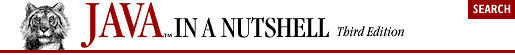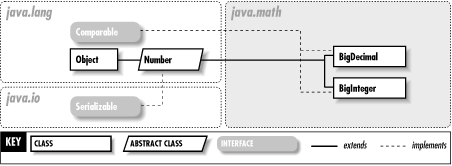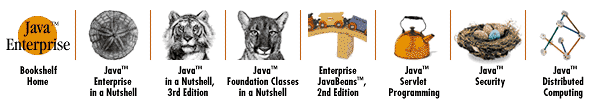home | O'Reilly's CD bookshelfs | FreeBSD | Linux | Cisco | Cisco Exam# Chapter 15. The java.math Package

The java.math package, new as of Java 1.1, contains classes for arbitrary-precision integer and floating-point arithmetic. Arbitrary-length integers are required for cryptography, and arbitrary-precision floating-point values are useful for financial applications that need to be careful about rounding errors. The class hierarchy of this extremely small package is shown in Figure 15-1.#### Figure 15-1. The java.math package

BigDecimalJava 1.1java.mathserializable comparable PJ1.1(opt)

This subclass of java.lang.Number represents a floating-point number of arbitrary size and precision. Its methods duplicate the functionality of the standard Java arithmetic operators. The compareTo() method compares the value of two BigDecimal objects and returns -1, 0, or 1 to indicate the result of the comparison.

A BigDecimal object is represented as an integer of arbitrary size and an integer scale that specifies the number of decimal places in the value. When working with BigDecimal values, you can explicitly specify the amount of precision (i.e., the number of decimal places) you are interested in. Also, whenever a BigDecimal method can discard precision (e.g., in a division operation), you are required to specify what sort of rounding should be performed on the digit to the left of the discarded digit or digits. The eight constants defined by this class specify the available rounding modes. Because the BigDecimal class provides arbitrary precision and gives you explicit control over rounding and the number of decimal places you are interested in, it can be useful when dealing with quantities that represent money or in other circumstances where the tolerance for rounding errors is low.

 public class BigDecimal extends Number implements Comparable { // Public Constructors public BigDecimal (BigInteger val); public BigDecimal (String val); public BigDecimal (double val); public BigDecimal (BigInteger unscaledVal, int scale); // Public Constants public static final int ROUND_CEILING ; =2 public static final int ROUND_DOWN ; =1 public static final int ROUND_FLOOR ; =3 public static final int ROUND_HALF_DOWN ; =5 public static final int ROUND_HALF_EVEN ; =6 public static final int ROUND_HALF_UP ; =4 public static final int ROUND_UNNECESSARY ; =7 public static final int ROUND_UP ; =0 // Public Class Methods public static BigDecimal valueOf (long val); public static BigDecimal valueOf (long unscaledVal, int scale); // Public Instance Methods public BigDecimal abs (); public BigDecimal add (BigDecimal val); public int compareTo (BigDecimal val); public BigDecimal divide (BigDecimal val, int roundingMode); public BigDecimal divide (BigDecimal val, int scale, int roundingMode); public BigDecimal max (BigDecimal val); public BigDecimal min (BigDecimal val); public BigDecimal movePointLeft (int n); public BigDecimal movePointRight (int n); public BigDecimal multiply (BigDecimal val); public BigDecimal negate (); public int scale (); public BigDecimal setScale (int scale); public BigDecimal setScale (int scale, int roundingMode); public int signum (); public BigDecimal subtract (BigDecimal val); public BigInteger toBigInteger (); 1.2 public BigInteger unscaledValue (); // Methods Implementing Comparable 1.2 public int compareTo (Object o); // Public Methods Overriding Number public double doubleValue (); public float floatValue (); public int intValue (); public long longValue (); // Public Methods Overriding Object public boolean equals (Object x); public int hashCode (); public String toString (); }

Hierarchy: Object-->Number(Serializable)-->BigDecimal(Comparable)

Passed To: Too many methods to list.

Returned By: Too many methods to list.

Type Of: org.omg.CORBA.FixedHolder.value

BigIntegerJava 1.1java.mathserializable comparable PJ1.1(opt)

This subclass of java.lang.Number represents integers that can be arbitrarily large (i.e., integers that are not limited to the 64 bits available with the long data type). BigInteger defines methods that duplicate the functionality of the standard Java arithmetic and bit-manipulation operators. The compareTo() method compares two BigInteger objects and returns -1, 0, or 1 to indicate the result of the comparison. The gcd(), modPow(), modInverse(), and isProbablePrime() methods perform advanced operations and are used primarily in cryptographic and related algorithms.

 public class BigInteger extends Number implements Comparable { // Public Constructors public BigInteger (String val); public BigInteger (byte[ ] val); public BigInteger (String val, int radix); public BigInteger (int signum, byte[ ] magnitude); public BigInteger (int numBits, java.util.Random rnd); public BigInteger (int bitLength, int certainty, java.util.Random rnd); // Public Constants public static final BigInteger ONE ; public static final BigInteger ZERO ; // Public Class Methods public static BigInteger valueOf (long val); // Public Instance Methods public BigInteger abs (); public BigInteger add (BigInteger val); public BigInteger and (BigInteger val); public BigInteger andNot (BigInteger val); public int bitCount (); public int bitLength (); public BigInteger clearBit (int n); public int compareTo (BigInteger val); public BigInteger divide (BigInteger val); public BigInteger[ ] divideAndRemainder (BigInteger val); public BigInteger flipBit (int n); public BigInteger gcd (BigInteger val); public int getLowestSetBit (); public boolean isProbablePrime (int certainty); public BigInteger max (BigInteger val); public BigInteger min (BigInteger val); public BigInteger mod (BigInteger m); public BigInteger modInverse (BigInteger m); public BigInteger modPow (BigInteger exponent, BigInteger m); public BigInteger multiply (BigInteger val); public BigInteger negate (); public BigInteger not (); public BigInteger or (BigInteger val); public BigInteger pow (int exponent); public BigInteger remainder (BigInteger val); public BigInteger setBit (int n); public BigInteger shiftLeft (int n); public BigInteger shiftRight (int n); public int signum (); public BigInteger subtract (BigInteger val); public boolean testBit (int n); public byte[ ] toByteArray (); public String toString (int radix); public BigInteger xor (BigInteger val); // Methods Implementing Comparable 1.2 public int compareTo (Object o); // Public Methods Overriding Number public double doubleValue (); public float floatValue (); public int intValue (); public long longValue (); // Public Methods Overriding Object public boolean equals (Object x); public int hashCode (); public String toString (); }

Hierarchy: Object-->Number(Serializable)-->BigInteger(Comparable)

Passed To: Too many methods to list.

Returned By: Too many methods to list.

Type Of: BigInteger.{ONE, ZERO}, java.security.spec.RSAKeyGenParameterSpec.{F0, F4}